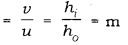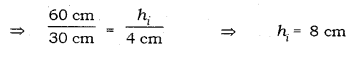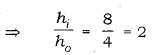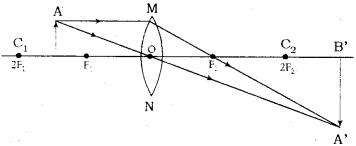# An object of height 4.0 cm is placed at a distance of 30 cm form the optical centre

An object of height 4.0 cm is placed at a distance of 30 cm form the optical centre ‘O’ of a convex lens of focal length 20 cm. Draw a ray diagram to find the position and size of the image formed. Mark optical centre ‘O’ and principal focus ‘F’ on the diagram. Also find the approximate ratio of size of the image to the size of the object.

Given data: u = - 30 cm; f = + 20 cm; h = 4 cm; v = ?; h = ?Magnification ratio:Ratio of size of image to size of object